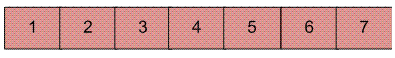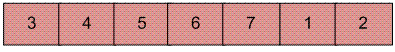# Python Program for Reversal algorithm for array rotation

Write a function rotate(arr[], d, n) that rotates arr[] of size n by d elements.

Example :

```Input :  arr[] = [1, 2, 3, 4, 5, 6, 7]
d = 2
Output : arr[] = [3, 4, 5, 6, 7, 1, 2]
```Rotation of the above array by 2 will make array## Recommended: Please solve it on “PRACTICE ” first, before moving on to the solution.

 `# Python program for reversal algorithm of array rotation ` ` `  `# Function to reverse arr[] from index start to end ` `def` `rverseArray(arr, start, end): ` `    ``while` `(start < end): ` `        ``temp ``=` `arr[start] ` `        ``arr[start] ``=` `arr[end] ` `        ``arr[end] ``=` `temp ` `        ``start ``+``=` `1` `        ``end ``=` `end``-``1` ` `  `# Function to left rotate arr[] of size n by d ` `def` `leftRotate(arr, d): ` `    ``n ``=` `len``(arr) ` `    ``rverseArray(arr, ``0``, d``-``1``) ` `    ``rverseArray(arr, d, n``-``1``) ` `    ``rverseArray(arr, ``0``, n``-``1``) ` ` `  `# Function to print an array ` `def` `printArray(arr): ` `    ``for` `i ``in` `range``(``0``, ``len``(arr)): ` `        ``print` `(arr[i]) ` ` `  `# Driver function to test above functions ` `arr ``=` `[``1``, ``2``, ``3``, ``4``, ``5``, ``6``, ``7``] ` `leftRotate(arr, ``2``) ``# Rotate array by 2 ` `printArray(arr) ` ` `  `# This code is contributed by Devesh Agrawal `

Output :

`3 4 5 6 7 1 2`

Time Complexity : O(n)

Please refer complete article on Reversal algorithm for array rotation for more details!

My Personal Notes arrow_drop_up

Improved By : rachitggupta2

Article Tags :

1

Please write to us at contribute@geeksforgeeks.org to report any issue with the above content.